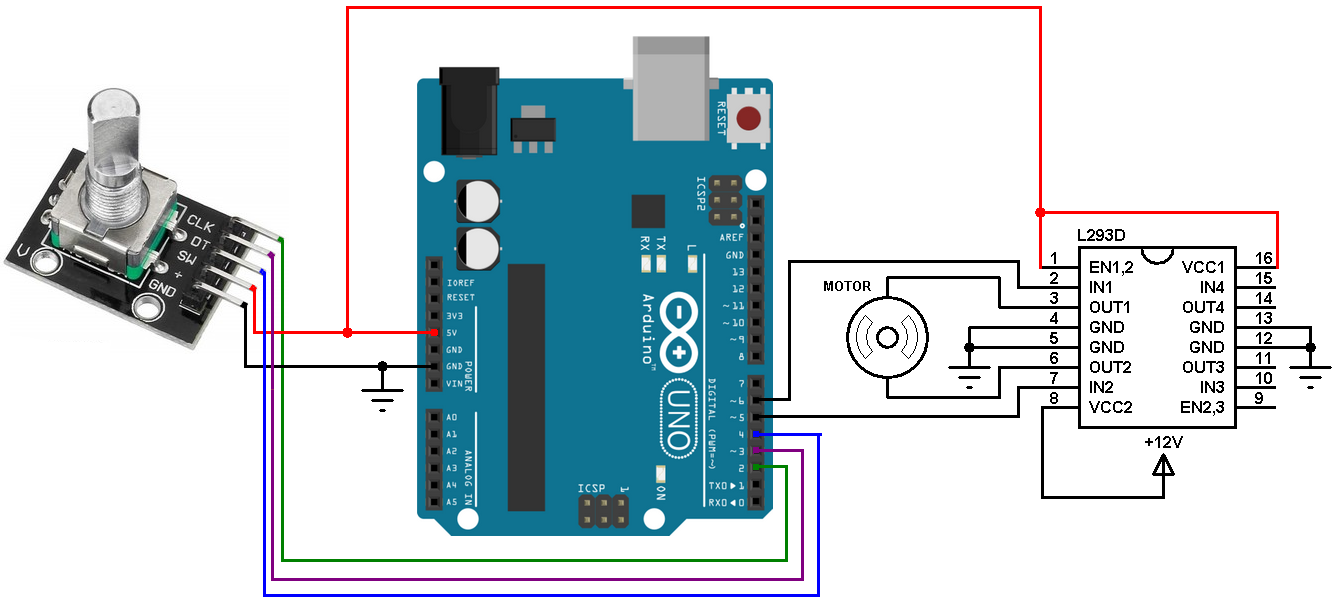# PWM dimmer LED Android

Who know one code on android mode to dimmer led to arduino bluetooth ?

See this code:

Thanks Noel
for the moment my problem is:
Remember the code of the circle with degrees and steps, which is moved by an encoder?
here the problem is that the code when it runs on pc
in java mode it works well while in android mode the same code of the circle does the flickering
would you know how i could fix it?
you see the code in old places
thanks

Do you mean this code by @jeremydouglass and @raron ?
I tested just with one knob and didn’t see any flickering.

``````// Rotary Encoder demo
// Code by @raron and @kerremydouglass

HwRotaryEncoder enc;
float scrollWheel = 0.0;

void setup() {
fullScreen();
enc = new HwRotaryEncoder(width/2, height/2, width/3, 8);
}

void draw() {
background(0, 0, 80);
fill(0);
enc.getDirection();
enc.update();
enc.display();
}

class HwRotaryEncoder {
PVector center;
PVector startDragPos;
PVector currentPos;
float encSize, slice;
float rotation, oldRotation;
int direction;
boolean encA, encB, oldEncA, oldEncB;
boolean active, mouseButtonWasPressed;
color knobColor;

HwRotaryEncoder(float w, float h, float encSize, int stepsPerRev) {
this.center       = new PVector(w, h);
this.startDragPos = new PVector(0, w);
this.currentPos   = new PVector(0, w);
this.encSize      = encSize;
this.direction    = 0;
this.rotation     = 0;
this.oldRotation  = 0;
this.oldEncA      = false;
this.oldEncB      = false;
this.active       = false;
}

boolean mouseInside() {
if (currentPos.mag() < encSize) return true;
return false;
}

void update() {
currentPos = new PVector(mouseX, mouseY).sub(center);
if (mousePressed == false) {
// a mouse button is not pressed
if (active) {
active = false;
oldRotation = rotation;
}
mouseButtonWasPressed = false;
} else {
// a mouse button is pressed
if (mouseButtonWasPressed == false) {
mouseButtonWasPressed = true;
if (mouseInside()) {
active = true;
startDragPos = new PVector(mouseX, mouseY).sub(center);
oldEncA = false;
oldEncB = false;
direction = 0;
}
}
}

// Mouse drag rotation
// If moving mouse fast, will probably skip steps or count backwards
if (active) {
if (rotation<0)      rotation += TWO_PI;
if (rotation>TWO_PI) rotation -= TWO_PI;

// Make rotary encoder quadrature pattern
if (pa == 1) encA = true;
else encA = false;
if (pb == 1) encB = true;
else encB = false;

// Count when transitioning pattern edges
if (encB & (encA ^ oldEncA)) {
if (encA) direction = 1;
else direction = -1;
}
if (!encB & (encA ^ oldEncA)) {
if (encA) direction = -1;
else direction = 1;
}
if (encA & (encB ^ oldEncB)) {
if (encB) direction = -1;
else direction = 1;
}
if (!encA & (encB ^ oldEncB)) {
if (encB) direction = 1;
else direction = -1;
}
oldEncA = encA;
oldEncB = encB;
}
}
}

int getDirection() {
int temp = direction;
direction = 0;
return temp;
}

void display() {
if (mouseInside() || active) knobColor = color(210);
else knobColor = color(192);
pushMatrix();
pushStyle();
translate(center.x, center.y);
stroke(255);
for (int i=0; i < quadsteps; i++) {
if (i == 0) strokeWeight(6);
else strokeWeight(3);
line( encSize*0.9, 0, encSize, 0 );
rotate(slice);
}
fill(knobColor);
ellipse(0, 0, encSize*6/8, encSize*6/8);
rotate(rotation);
stroke(0);
strokeWeight(6);
line( 0, 0, encSize*6/8, 0 );
popMatrix();
popStyle();
}
}
``````

Noel
Tankyou for the code i evening try ti run
It’s have one sketch for arduino ?
Hello

You didn’t say what it is for. Rotation of a stepper motor? What resolution?

DC Motor
yes i have 2 encoder
1: is 200 step for revolution
or
1: 1600 step for revolution
Conncect wire:
+A B -
Tankyou

OK, but where is your Arduino code?

this my code:

``````volatile long temp, counter = 0; //This variable will increase or decrease depending on the rotation of encoder

void setup() {
Serial.begin (9600);

pinMode(2, INPUT_PULLUP); // internal pullup input pin 2

pinMode(3, INPUT_PULLUP); // internalเป็น pullup input pin 3
//Setting up interrupt
//A rising pulse from encodenren activated ai0(). AttachInterrupt 0 is DigitalPin nr 2 on moust Arduino.
attachInterrupt(0, ai0, RISING);

//B rising pulse from encodenren activated ai1(). AttachInterrupt 1 is DigitalPin nr 3 on moust Arduino.
attachInterrupt(1, ai1, RISING);
}

void loop() {
// Send the value of counter
if( counter != temp ){
Serial.println (counter);
temp = counter;
}
}

void ai0() {
// ai0 is activated if DigitalPin nr 2 is going from LOW to HIGH
// Check pin 3 to determine the direction
counter++;
}else{
counter--;
}
}

void ai1() {
// ai0 is activated if DigitalPin nr 3 is going from LOW to HIGH
// Check with pin 2 to determine the direction
counter--;
}else{
counter++;
}
}
``````

when i move encoder the circle this code by @jeremydouglass and @raron not move

Is it something like this?Hello Noel
No my circuit is some i use mega 2560
Encoder os digitale encoder : share channel A is Pin and channel B is Pin 3
And driver motor is some you schematic

Hello Noel
No my circuit is some i use mega 2560
Encoder os digitale encoder : where channel A is Pin 2 and channel B is Pin 3
And driver motor is some you schematic

Can you give me the name of the driver board?

driver motor BTS7960 43A

its some but i have arduino 2560 and i use pin forward 13,bakward 11, encoder to 2,and 3I’m lost here. As far as I can see, the BTS7960 43A is pulse-width modulated where rotation can be reversed and speed controlled. Anyway I have some code for a stepper motor where you can set the exact angle position, but you need a stepper pin and a direction pin.

I have a DC motor and not step by step, the position of the angle is given by the encoder

I don’t see how this can be done with a dc motor, because you don’t have reference points to ensure the right angle unless you have a dc motor with an encoder coupled to the axis.